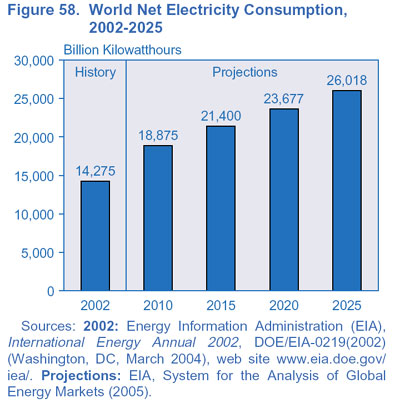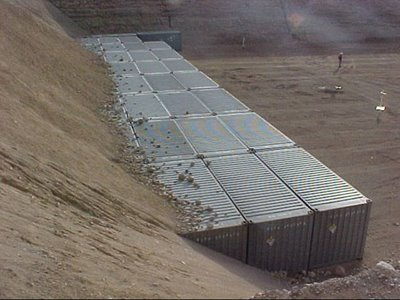# to Power the Whole World

I have claimed on here that thorium, if efficiently utilized in a liquid-fluoride reactor, is an energy source of such magnitude that it is not difficult to conceive of an entire planet powered by thorium.

## The Calculation

So let me lay out the calculations upon which I base my argument. In a fission reaction, thorium-232 (having been transmuted to uranium-233) will release roughly 190 MeV of energy per fission reaction. Assuming that the original thorium had a mass of 232 atomic units (u), then that is equivalent to 190 MeV/232 u = 820 keV/u.

How much energy is that? If converted to electricity at 50% efficiency (which can be achieved through the use of a helium gas turbine power conversion system), 820 keV/u is equivalent to 11 billion kilowatt-hours per metric ton of thorium. (Note that a billion kilowatt-hours [BKWH] is equivalent to a terawatt-hour [TWH].)In 2003, it was estimated that the world produced 16.5 trllion kilowatt-hours of electricity. If this had all been produced by liquid-fluoride thorium reactors, this would have required 1500 metric tonnes of thorium. Future energy projections foresee electrical production reaching 21.4 trillion kilowatt-hours by 2015. To bring the entire world’s population up to the level of the average American’s electrical consumption would require 80 trillion kilowatt-hours.

Is 1500 metric tonnes a lot? Well, consider that until recently, the United States had 3216 metric tonnes of thorium nitrate in storage. Recently, this thorium was deemed worthless by the government and buried at the Nevada Test Site. Thorium is a very dense material, and 1500 metric tonnes of thorium metal would only occupy 130 cubic meters of volume, or about the volume of a room 23 ft on a side and 9 feet high.## How does Uranium Compare?

How does this compare to conventional (solid-core uranium) nuclear? In 2002, the world’s nuclear reactors produced 2.56 trillion kilowatt-hours of electricity and consumed 67,000 metric tonnes of uranium to do it. That is equivalent to only 0.038 billion kilowatt-hours per metric tonne of uranium. Why such a disparity between thorium at 11 TWH/MT and uranium at 0.038 TWH/MT? Because today’s nuclear only utilizes the small (0.7%) amount of uranium that is fissile (U-235), and only ends up using about half of that. Light-water reactors burning scarce U-235 and converting it to electricity at 33% efficiency are just less efficient than liquid-fluoride thorium reactors at 50% efficiency.

It is worth considering for a moment that the thorium required to fuel the entire world’s electrical needs would fit in a reasonably sized room, and the thorium required would only be about 2% of the mass of uranium mined today.